## A power learning aid combining Coaching Calculators, Practice and Guide to help students master linear equations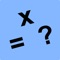# 20/20 Linear Equations

by Walter Kissach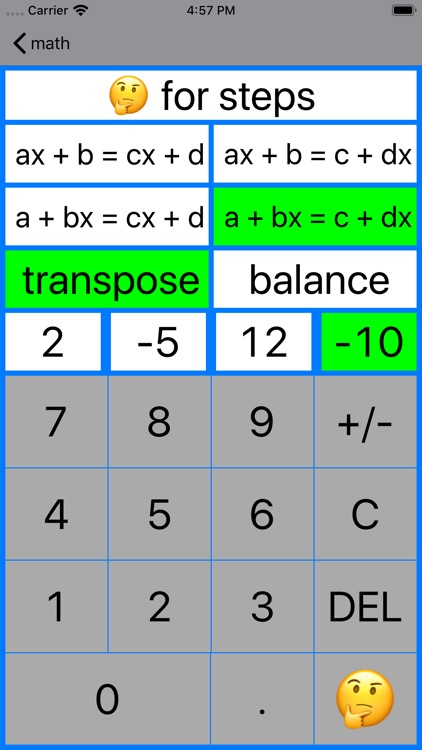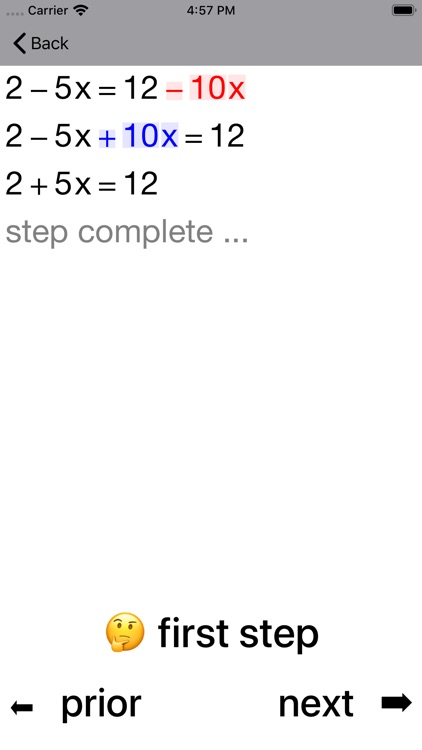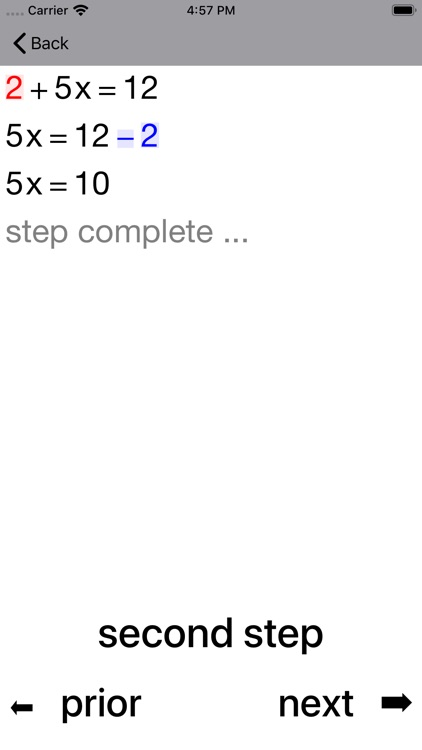A power learning aid combining Coaching Calculators, Practice and Guide to help students master linear equations.### App Details

Version
6.0
Rating
(1)
Size
10Mb
Genre
Education Reference
Last updated
March 19, 2020
Release date
May 8, 2009

### App Screenshots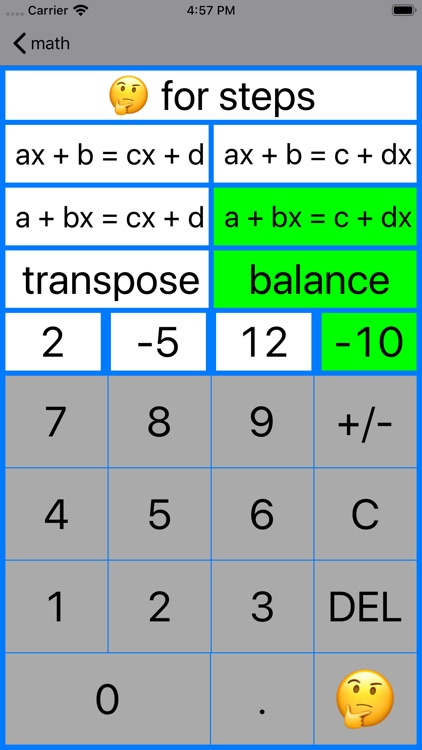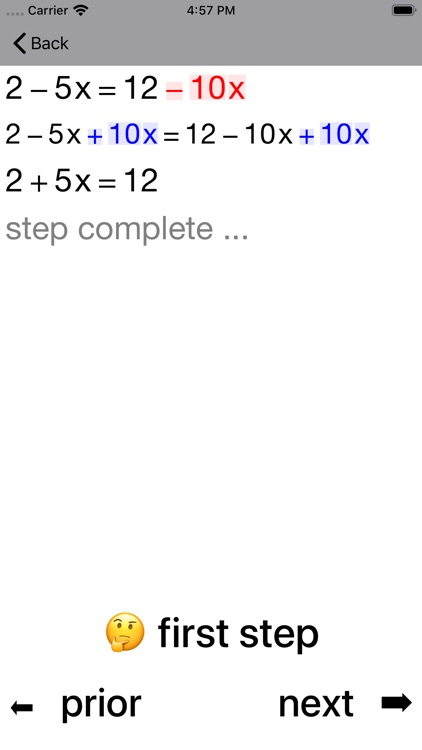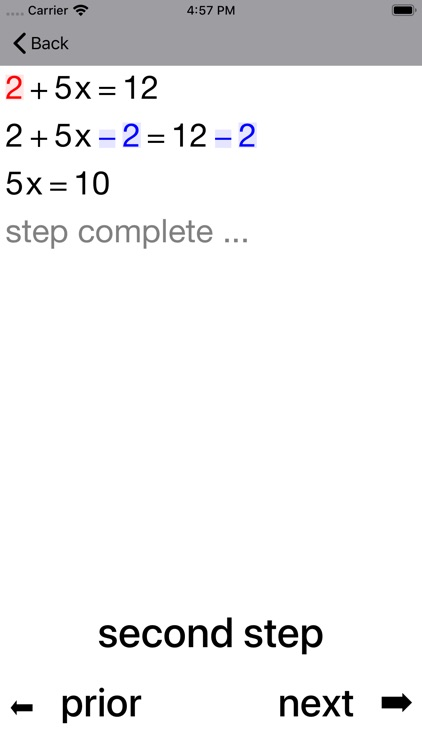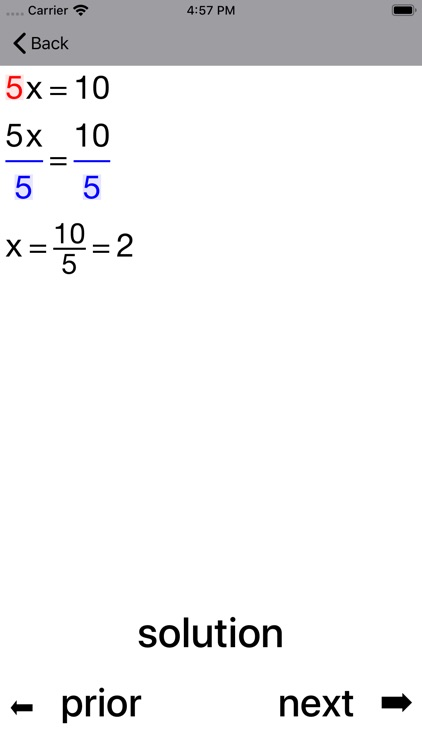### App Store Description

A power learning aid combining Coaching Calculators, Practice and Guide to help students master linear equations.

Coaching Calculators focus on one-step, two-step and multi-step equations such as x + 6 = 9, x/2 − 5 = 6 and 5x + 2 = 3x + 8 respectively.

One-step
x − 2 = 3, 5 + x = 4, 3x = -12, x/7 = 2

Two-step
3x + 8 = 20, x/9 + 2 = 5

Multi-step
4 + 9x = 2x + 18

The Practice unit focuses on equations with parentheses and fractions along with one-step, two-step and multi-step equations.

Equations with parentheses and fractions
10 − 3(2 − 3x) = 2x + 9
(1/2)(2x − 5) = 3x + 3/4

The step by step Guide includes many worked examples that highlight and simplify algebra that students find hard and. The Guide looks at Cross Multiplication as a useful shortcut

Equations with fractions
(5/6)x − (3/4)x = 7/3

Equations with decimals
0.25x = 9.9 − 0.2x

Students learn quickly as they work with the Coaching Calculator to review each step of the process in detail.

Disclaimer:
AppAdvice does not own this application and only provides images and links contained in the iTunes Search API, to help our users find the best apps to download. If you are the developer of this app and would like your information removed, please send a request to [email protected] and your information will be removed.